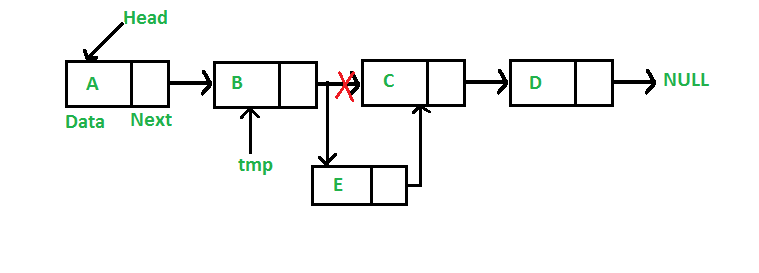$$\newcommand{\vecs}{\overset { \rightharpoonup} {\mathbf{#1}} }$$ $$\newcommand{\vecd}{\overset{-\!-\!\rightharpoonup}{\vphantom{a}\smash {#1}}}$$$$\newcommand{\id}{\mathrm{id}}$$ $$\newcommand{\Span}{\mathrm{span}}$$ $$\newcommand{\kernel}{\mathrm{null}\,}$$ $$\newcommand{\range}{\mathrm{range}\,}$$ $$\newcommand{\RealPart}{\mathrm{Re}}$$ $$\newcommand{\ImaginaryPart}{\mathrm{Im}}$$ $$\newcommand{\Argument}{\mathrm{Arg}}$$ $$\newcommand{\norm}{\| #1 \|}$$ $$\newcommand{\inner}{\langle #1, #2 \rangle}$$ $$\newcommand{\Span}{\mathrm{span}}$$ $$\newcommand{\id}{\mathrm{id}}$$ $$\newcommand{\Span}{\mathrm{span}}$$ $$\newcommand{\kernel}{\mathrm{null}\,}$$ $$\newcommand{\range}{\mathrm{range}\,}$$ $$\newcommand{\RealPart}{\mathrm{Re}}$$ $$\newcommand{\ImaginaryPart}{\mathrm{Im}}$$ $$\newcommand{\Argument}{\mathrm{Arg}}$$ $$\newcommand{\norm}{\| #1 \|}$$ $$\newcommand{\inner}{\langle #1, #2 \rangle}$$ $$\newcommand{\Span}{\mathrm{span}}$$$$\newcommand{\AA}{\unicode[.8,0]{x212B}}$$

Sometimes we have a linked list and we need to insert a node somewhere other than at the end of the list. We will take a look at a couple of different ways to insert a node into an existing list

A new node can be added to a linked list in one of three ways
1) At the front of the linked list
2) After a given node.
3) At the end of the linked list.

### Add a node at the front

In this picture we reset the HEAD to point to the new node, E, and then allow the new node's Next field point to the previous HEAD, A.In this section all nodes have the format:

// A linked list node
class Node
{
public:
char data;
Node *next;
};


There is a four step process to insert a new node at the head of the linked list:

1. Allocate memory for the new node
2. Put our data into the new node
3. Set Next of the new node to point to the previous Head
4. Reset Head to point to the new node

Here is the code for our push() function:

/* Given a reference (pointer to pointer)
to the head of a list and a char,
inserts a new node on the front of the list. */
{
/* 1. allocate node */
Node* new_node = new Node();
/* 2. put in the data */
new_node->data = new_data;
/* 3. Make next of new node point to previous head */
/* 4. move the head to point to the new node */
} 

This code performs our 4 steps:

1. allocate memory for a new node called new_node (you can call it whatever you want)
2. we insert the data, that was passed in as an argument, into the data field of the Node we just created
3. take the head_ref pointer, that points to the original head, and set the next field of the new node to point at the previous head
4. take the address of the new_node and reset the head_ref to point to this new node as the head of the linked list

### Add a node after a given node:

As noted above, we can insert a node after a specific node. We are given pointer to a node, and the new node is inserted after the given node.// Given a node prev_node, insert a
// new node after the given
// prev_node
void insertAfter(Node* prev_node, char new_data)
{
// 1. Check if the given prev_node is NULL
if (prev_node == NULL)
{
cout << "the given previous node cannot be NULL";
return;
}

// 2. Allocate new node
Node* new_node = new Node();

// 3. Put in the data
new_node->data = new_data;

// 4. Make next of new node as next of prev_node
new_node->next = prev_node->next;

// 5. move the next of prev_node as new_node
prev_node->next = new_node;
} 

In this code the push() function is called with 2 arguments, the previous node, prev_node, and the data, new_data, for the new node. The code inserts the new node AFTER the previous node that is passed in. This function fails if the prev_node is NULL, if the prev_node is NULL, then something is wrong. We cannot insert a new node if we do not know where the previous node is. NOTICE: this is saying the NODE is NULL....NOT the prev_node->next. If we have a node where prev_node is NULL then we are at the end of the linked list, and we can insert a new node at the end of the list.

### Add a node at the end:

The new node is added after the last node of the given Linked List. For example if the given Linked List is 5->10->15->20->25 and we add an item 30 at the end, then the Linked List becomes 5->10->15->20->25->30.

It is possible to insert a new node at the end of the linked list using the previous methodology. However, if we are given the head pointer, then we must traverse the linked list until we get the the end of the list. Once we find the end of the linked list - that is when the prev_node->next is NULL, then change the next of this last node to point to the new node, and set the next of the new node to NULL.Here is the code for the above image. The Head is the address of the first node, the Next member is the memory address of the next node in the list.

Most of this code we have seen before, and should be at least familiar. This code is a good example of traversing the list, which is simply done be a short while loop. Step #5 shows how easy it is to walk across the list. The while loop condition looks at the Next member of the class, if it is set to NULL then we are at the end of the list. If the Next member is NOT NULL, we set the pointer last to the last->next then go back to the while condition and checks for NULL again.

// Given a reference (pointer to pointer) to the head
// of a list and an char, appends a new node at the end
{

// 1. allocate node
Node* new_node = new Node();

// Used in step 5

// 2. Put in the data
new_node->data = new_data;

// 3. This new node is going to be the last node, so make next of it as NULL
new_node->next = NULL;

// 4. If the Linked List is empty, then make the new node as head
{
return;
}

// 5. Else traverse till the last node
while (last->next != NULL)
last = last->next;

// 6. Change the next of last node
last->next = new_node;
return;
}